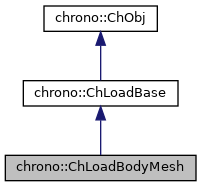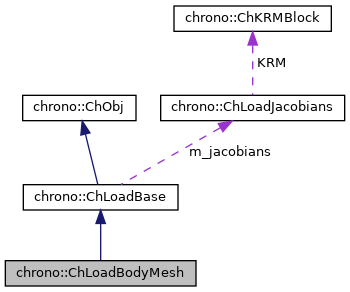## Description

Class for applying loads to a triangle mesh belonging to a ChBody, as a cluster of forces operating on the underlying rigid body.

It is useful for doing cosimulation: one can pass this object's vertex & faces to an external software (ex. CFD) that in turn will perform collision detection with its entities, compute forces, send forces back to Chrono via this object. Note, this is based on a cluster of std::vector<std::shared_ptr<ChLoadBodyForce>>, but the class itself could bypass all methods of ChLoadBodyForce and directly implement a more efficient LoadIntLoadResidual_F.

#include <ChLoadBodyMesh.h>[legend][legend]

## Public Member Functions

ChLoadBodyMesh (std::shared_ptr< ChBody > cbody, geometry::ChTriangleMeshConnected &cmesh)

"Virtual" copy constructor (covariant return type).

void OutputSimpleMesh (std::vector< ChVector<>> &vert_pos, std::vector< ChVector<>> &vert_vel, std::vector< ChVector< int >> &triangles)
Get the collision mesh where vertex are given in a vector of xyz points, expressed in absolute coordinates, and triangles are given as indexes to the three vertexes in that vector (similar to Wavefront OBJ meshes). More...

void InputSimpleForces (const std::vector< ChVector<>> vert_forces, const std::vector< int > vert_ind)
Set the forces to the body, where forces are given as a vector of xyz vectors (expressed in absolute coordinates) and indexes to the referenced vertex, as obtained by OutputSimpleMesh. More...

void SetContactMesh (geometry::ChTriangleMeshConnected &mmesh)
Set the contact mesh (also resets the applied nodes).

geometry::ChTriangleMeshConnectedGetContactMesh ()
Get the contact mesh.

std::vector< std::shared_ptr< ChLoadBodyForce > > & GetForceList ()
Access the list of applied forces, to allow adding new ones, removing them, counting them, etc. More...

Gets the number of DOFs affected by this load (position part)

Gets the number of DOFs affected by this load (speed part)

virtual void LoadGetStateBlock_x (ChState &mD) override
Gets all the current DOFs packed in a single vector (position part)

virtual void LoadGetStateBlock_w (ChStateDelta &mD) override
Gets all the current DOFs packed in a single vector (speed part)

virtual void LoadStateIncrement (const ChState &x, const ChStateDelta &dw, ChState &x_new) override
Increment a packed state (ex. More...

Number of coordinates in the interpolated field, ex=3 for a tetrahedron finite element or a cable, = 1 for a thermal problem, etc.

virtual void ComputeQ (ChState *state_x, ChStateDelta *state_w) override
Compute Q, the generalized load. More...

virtual void ComputeJacobian (ChState *state_x, ChStateDelta *state_w, ChMatrixRef mK, ChMatrixRef mR, ChMatrixRef mM) override
Compute jacobians. More...

virtual bool IsStiff () override
Report if this is load is stiff. More...

virtual void CreateJacobianMatrices () override
Create the jacobian loads if needed, and also set the ChVariables referenced by the sparse KRM block.

Adds the internal loads Q (pasted at global nodes offsets) into a global vector R, multiplied by a scaling factor c, as R += forces * c.

Increment a vector R with a term that has M multiplied a given vector w: R += c*M*w also R += c*(-dQ/da)*w Not needed (ex. More...

virtual void InjectKRMmatrices (ChSystemDescriptor &mdescriptor) override
Tell to a system descriptor that there are item(s) of type ChKblock in this object (for further passing it to a solver)

virtual void KRMmatricesLoad (double Kfactor, double Rfactor, double Mfactor) override
Adds the current stiffness K and damping R and mass M matrices in encapsulated ChKblock item(s), if any. More...Public Member Functions inherited from chrono::ChLoadBase
Access the jacobians (if any, i.e. if this is a stiff load)

virtual void Update (double time)
Update: this is called at least at each time step. More...Public Member Functions inherited from chrono::ChObj
ChObj (const ChObj &other)

int GetIdentifier () const
Gets the numerical identifier of the object.

void SetIdentifier (int id)
Sets the numerical identifier of the object.

double GetChTime () const
Gets the simulation time of this object.

void SetChTime (double m_time)
Sets the simulation time of this object.

const char * GetName () const
Gets the name of the object as C Ascii null-terminated string -for reading only!

void SetName (const char myname[])
Sets the name of this object, as ascii string.

std::string GetNameString () const
Gets the name of the object as C Ascii null-terminated string.

void SetNameString (const std::string &myname)
Sets the name of this object, as std::string.

void MFlagsSetAllOFF (int &mflag)

void MFlagsSetAllON (int &mflag)

void MFlagSetON (int &mflag, int mask)

void MFlagSetOFF (int &mflag, int mask)

int MFlagGet (int &mflag, int mask)

virtual void ArchiveOUT (ChArchiveOut &marchive)
Method to allow serialization of transient data to archives.

virtual void ArchiveIN (ChArchiveIn &marchive)
Method to allow de-serialization of transient data from archives.

virtual std::string & ArchiveContainerName ()Protected Attributes inherited from chrono::ChLoadBaseProtected Attributes inherited from chrono::ChObj
double ChTime
the time of simulation for the object

## ◆ ComputeJacobian()

 void chrono::ChLoadBodyMesh::ComputeJacobian ( ChState * state_x, ChStateDelta * state_w, ChMatrixRef mK, ChMatrixRef mR, ChMatrixRef mM )
overridevirtual

Compute jacobians.

Not needed when forces are constant, btw.

Parameters
 state_x state position to evaluate jacobians state_w state speed to evaluate jacobians mK result dQ/dx mR result dQ/dv mM result dQ/da

## ◆ ComputeQ()

 void chrono::ChLoadBodyMesh::ComputeQ ( ChState * state_x, ChStateDelta * state_w )
overridevirtual

Parameters
 state_x state position to evaluate Q state_w state speed to evaluate Q

## ◆ GetForceList()

inline

Access the list of applied forces, to allow adding new ones, removing them, counting them, etc.

Note that only nodes from the reference mesh should be added.

## ◆ InputSimpleForces()

 void chrono::ChLoadBodyMesh::InputSimpleForces ( const std::vector< ChVector<>> vert_forces, const std::vector< int > vert_ind )

Set the forces to the body, where forces are given as a vector of xyz vectors (expressed in absolute coordinates) and indexes to the referenced vertex, as obtained by OutputSimpleMesh.

NOTE: do not insert/remove nodes from the collision mesh between the OutputSimpleMesh-InputSimpleForces pair!

Parameters
 vert_forces array of forces (in absolute frame) vert_ind indexes of vertices with applied forces

## ◆ IsStiff()

inlineoverridevirtual

Report if this is load is stiff.

If so, InjectKRMmatrices will provide the jacobians of the load.

overridevirtual

Adds the current stiffness K and damping R and mass M matrices in encapsulated ChKblock item(s), if any.

The K, R, M matrices are added with scaling values Kfactor, Rfactor, Mfactor.

inlinevirtual

Increment a vector R with a term that has M multiplied a given vector w: R += c*M*w also R += c*(-dQ/da)*w Not needed (ex.

override to {} ) if no M is involved, ex. no inertial effects. a scaling factor

Parameters
 R result: the R residual, R += c*M*v w the w vector

 void chrono::ChLoadBodyMesh::LoadStateIncrement ( const ChState & x, const ChStateDelta & dw, ChState & x_new )
overridevirtual

Increment a packed state (ex.

as obtained by LoadGetStateBlock_x()) by a given packed state-delta. Compute: x_new = x + dw. Ex. this is called by the BDF numerical differentiation routine that computes jacobian in the default ComputeJacobian() fallback, if not overriding ComputeJacobian() with an analytical form.

## ◆ OutputSimpleMesh()

 void chrono::ChLoadBodyMesh::OutputSimpleMesh ( std::vector< ChVector<>> & vert_pos, std::vector< ChVector<>> & vert_vel, std::vector< ChVector< int >> & triangles )

Get the collision mesh where vertex are given in a vector of xyz points, expressed in absolute coordinates, and triangles are given as indexes to the three vertexes in that vector (similar to Wavefront OBJ meshes).

Note that indexes are 0-based. These vectors can be later sent to another computing node that computes, say, CFD forces on the mesh.

Parameters
 vert_pos array of vertexes (absolute xyz positions) vert_vel array of vertexes (absolute xyz velocities, might be useful) triangles array of triangles (indexes to vertexes, ccw)

The documentation for this class was generated from the following files: